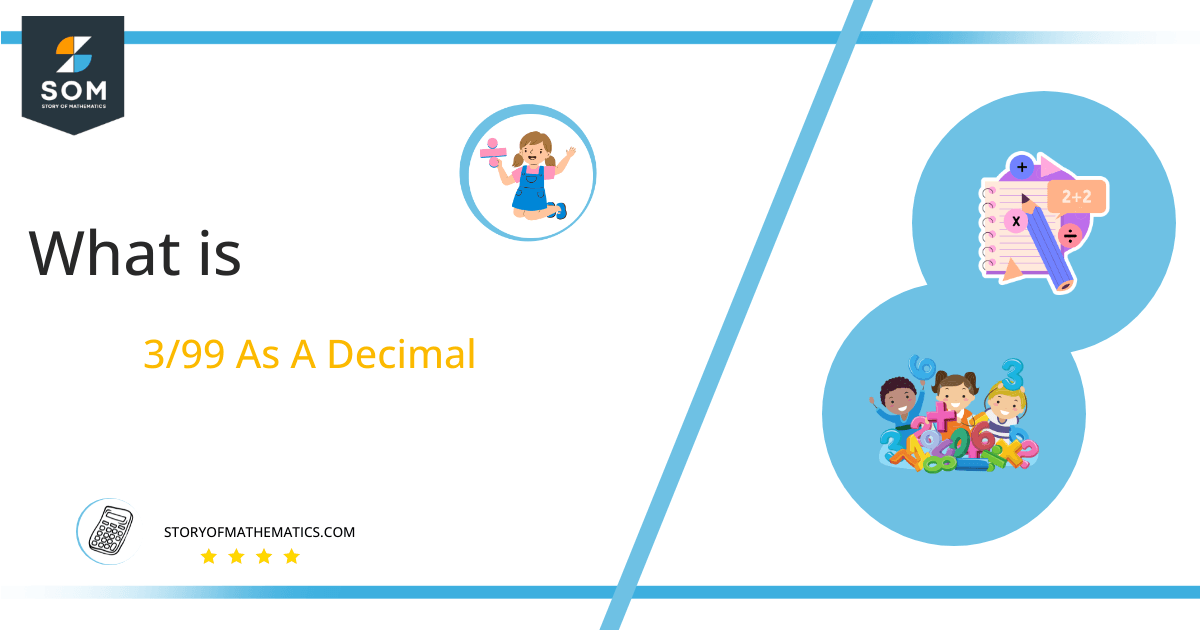# What Is 3/99 as a Decimal + Solution With Free Steps

The fraction 3/99 as a decimal is equal to 0.0303.

We know that Division is one of the four primary operators of mathematics and by applying the long division method we can convert the fractional form into its equivalent decimal form. The long division is easy to use as it breaks down the problem into a sequence of easier steps.Here, we are more interested in the division types that result in a Decimal value, as this can be expressed as a Fraction. We see fractions as a way of showing two numbers having the operation of Division between them that result in a value that lies between two Integers.

Now, we introduce the method used to solve said fraction to decimal conversion, called Long Division, which we will discuss in detail moving forward. So, let’s go through the Solution of fraction 3/99.

## Solution

First, we convert the fraction components, i.e., the numerator and the denominator, and transform them into the division constituents, i.e., the Dividend and the Divisor, respectively.

This can be done as follows:

Dividend = 3

Divisor = 99

Now, we introduce the most important quantity in our division process: the Quotient. The value represents the Solution to our division and can be expressed as having the following relationship with the Division constituents:

Quotient = Dividend $\div$ Divisor = 3 $\div$ 99

This is when we go through the Long Division solution to our problem. The solution for fraction 3/99 is shown in the following figure.Figure 1

## 3/99 Long Division Method

We start solving a problem using the Long Division Method by first taking apart the division’s components and comparing them. As we have 3 and 99, we can see how 3 is Smaller than 99, and to solve this division, we require that 3 be Bigger than 99.

This is done by multiplying the dividend by 10 and checking whether it is bigger than the divisor or not. If so, we calculate the Multiple of the divisor closest to the dividend and subtract it from the Dividend. This produces the Remainder, which we then use as the dividend later.

Since 3 when multiplied by 10 becomes 30 which is still smaller than 99. Therefore we will multiply 30 by 10 again and add a zero in the quotient after the decimal point. By doing this the dividend will become 300 which is bigger than 99 and hence divisible by 99.

Now, we begin solving for our dividend 300.

We take this 300 and divide it by 99; this can be done as follows:

300 $\div$ 99 $\approx$ 3

Where:

99 x 3 = 297

This will lead to the generation of a Remainder equal to 300 – 297 = 3. Now this means we have to repeat the process by Converting the 3 into 30.

However, 30 is still smaller than 99. Therefore we will multiply 30 by 10 again and add a zero in the quotient after the decimal point. By doing this the dividend will become 300 which is bigger than 99.

300 $\div$ 99 $\approx$ 3

Where:

99 x 3 = 297

Finally, we have a Quotient generated as 0.0303, with a Remainder equal to 3.Images/mathematical drawings are created with GeoGebra.